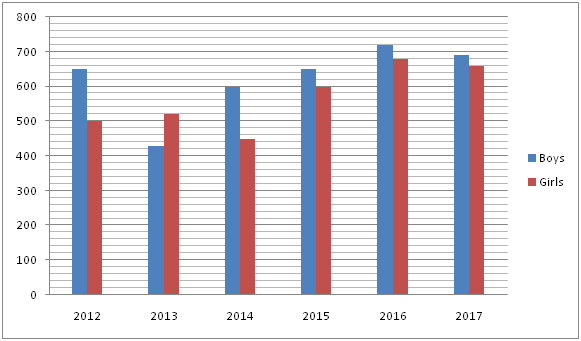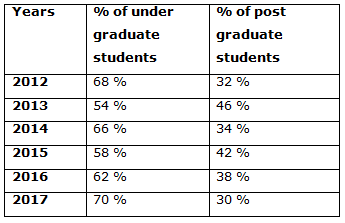# IBPS PO Prelims 2018 – Quantitative Aptitude Questions Day-2

Dear Readers, Bank Exam Race for the Year 2018 is already started, To enrich your preparation here we have providing new series of Practice Questions on Quantitative Aptitude – Section. Candidates those who are preparing for IBPS PO Prelims 2018 Exams can practice these questions daily and make your preparation effective.

[WpProQuiz 3955]

Directions (Q. 1 – 5): Study the following information carefully and answer the given questions:

The following bar graph shows the total number of girls and boys studied in a certain college in different years and the table shows the percentage distribution of total number of under graduate and post graduate students in that college in different years.1) Find the difference between the total number of girls to that of total number of boys studied in the college in all the given years together?

a) 560

b) 420

c) 330

d) 270

e) None of these

2) Find the ratio between the total number of under graduate students studied in the year 2012 to that of total number of post graduate students studied in the year 2014?

a) 782: 357

b) 157: 52

c) 289: 113

d) 354: 95

e) None of these

3) Find the average number of post graduate students studied in the year 2012, 2015 and 2016 together?

a) 520

b) 440

c) 452

d) 475

e) None of these

4) Total number of post graduate students studied in the year 2013 is approximately what percentage of total number of girls studied in the year 2012 and 2015 together?

a) 50 %

b) 40 %

c) 25 %

d) 65 %

e) 75 %

5) Total number of boys studied in the year 2015 is approximately what percentage more/less than the total number of undergraduate students studied in the year 2014?

a) 6 % less

b) 15 % more

c) 6 % more

d) 22 % less

e) 15 % less

Directions (Q. 6 – 10): What approximate value should come in place of question mark (?) in the following questions?

6) (2/17) of 7649 + (3/11) of 15673 =? × 5

a) 1170

b) 1350

c) 1035

d) 1925

e) 2130

7) 32 % of 4399 + 282 ÷ 7 × 4.2 + 13.232 =?2

a) 45

b) 47

c) 39

d) 41

e) 37

8) 1249.72 ÷ 49.5 =? + 419.65 – (216 ÷ 5 × 12.3)

a) 150

b) 270

c) 230

d) 120

e) 310

9) (14/17) ÷ (310/186) × 622 + 1253.67 – 53 % of 2199 =?

a) 500

b) 400

c) 650

d) 250

e) 700

10) 47 % of 1299 + (7/14.78) of 6059 – 42 % of 899 =?

a) 3060

b) 2850

c) 2370

d) 1890

e) 1320

Direction (1-5) :

The total number of girls studied in the college in all the given years together

= > 500 + 520 + 450 + 600 + 680 + 660 = 3410

The total number of boys studied in the college in all the given years together

= > 650 + 430 + 600 + 650 + 720 + 690 = 3740

Required difference = 3740 – 3410 = 330

The total number of under graduate students studied in the year 2012

= > 1150*(68/100) = 782

The total number of post graduate students studied in the year 2014

= > 1050*(34/100) = 357

Required ratio = 782: 357

The total number of post graduate students studied in the year 2012, 2015 and 2016 together

= > 1150*(32/100) + 1250*(42/100) + 1400*(38/100)

= > 368 + 525 + 532 = 1425

Required average = 1425/3 = 475

Total number of post graduate students studied in the year 2013

= > 950*(46/100) = 437

Total number of girls studied in the year 2012 and 2015 together

= > 500 + 600 = 1100

Required % = (437/1100)*100 = 40 %

Total number of boys studied in the year 2015 = 650

Total number of undergraduate students studied in the year 2014

= > 1050*(66/100) = 693

Required % = [(693 – 650)/693]*100 = 6 % less

Direction (6-10) :

(2/17) of 7649 + (3/11) of 15673 =? × 5

(2/17)*7650 + (3/11)*15675 = 5x

900 + 4275 = 5x

5175 = 5x

X = 5175/5 = 1035

32 % of 4400 + 282 ÷ 7 × 4 + 132 = x2

X2 = (32/100)*4400 + (28*28*4)/7 + 169

X2 = 1408 + 448 + 169

X2 = 2025

X = 45

1250 ÷ 50 = x + 420 – (215 ÷ 5 × 12)

(1250/50) – 420 + [(215/5)*12] = x

X = 25 – 420 + 516 = 121

X = 120

(14/17) ÷ (311/187) × 622 + 1254 – 53 % of 2200 = x

(14/17)*(187/311)*622 + 1254 – (53/100)*2200 = x

X = 308 + 1254 – 1166 = 396

X = 400

47 % of 1300 + (7/15) of 6060 – 42 % of 900 = x

X = (47/100)*1300 + (7/15)*6060 – (42/100)*900

X = 611 + 2828 – 378 = 3061

X = 3060

Daily Practice Test Schedule | Good Luck

 Topic Daily Publishing Time Daily News Papers & Editorials 8.00 AM Current Affairs Quiz 9.00 AM Current Affairs Quiz (Hindi) 9.30 AM IBPS PO Prelims – Reasoning 10.00 AM IBPS PO Prelims – Quantitative Aptitude 11.00 PM Vocabulary (Based on The Hindu) 12.00 PM IBPS PO Prelims – English Language 1.00 PM IBPS PO/Clerk – GK 3.00 PM Daily Current Affairs Updates 5.00 PM IBPS Clerk Prelims – Reasoning 6.00 PM IBPS Clerk Prelims – Reasoning (Hindi) 6.30 PM IBPS Clerk Prelims – Quantitative Aptitude 7.00 PM IBPS Clerk Prelims – Quantitative Aptitude (Hindi) 7.30 PM IBPS Clerk Prelims – English Language 8.00 PM